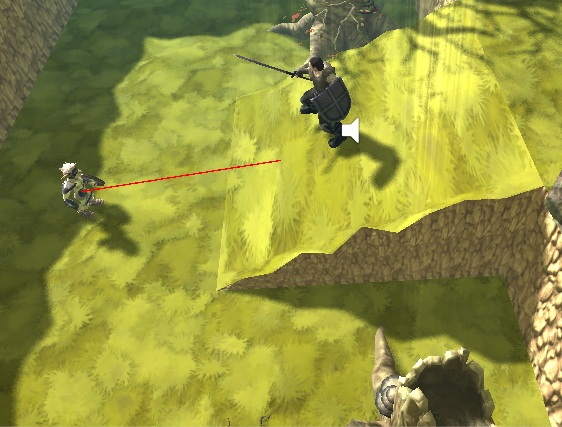# Raycast forward except for the Y axis

I need to Raycast forward from the enemy except for the Y axis which should point to the player

Basically I want the enemy raycast direction to be forward but also point to the players height, because when the player is on for example a ramp the enemy cant see it.

Here is an image is an image to illustrate the problem.This is the raycast function.

``````void FixedUpdate(){
sight.origin = new Vector3(transform.position.x, transform.position.y + 0.5f, transform.position.z);
sight.direction = transform.forward;
RaycastHit rayHit;
//Vector3 fwd = transform.TransformDirection(Vector3.forward);

if (Physics.Raycast(sight, out rayHit, chaseRange)){
Debug.DrawLine(sight.origin, rayHit.point, Color.red);
if (rayHit.collider.tag == "Player"){
CurrentState = EnemyStates.following;
}

if (rayHit.collider.tag != "Player" && rayHit.collider.tag != "Enemy"){
CurrentState = EnemyStates.idle;
}
}

}
``````

I’d flip it around - instead of saying “I want to raycast forward and see if I hit the player”, I’d say “I want to check whether the vector to the player is close to my forward vector.”

Something like this:

``````GameObject player = GameObject.FindGameObjectWithTag("Player");
Vector3 vectorToPlayer = player.transform.position - sight.origin;

// Calculate the vectorToPlayer as if it were flat and unit-length
Vector3 flatDirToPlayer =
new Vector3(vectorToPlayer.x, 0, vectorToPlayer.z).normalized;

// Check the angle is within 5 degrees
if(Vector3.Angle(flatDirToPlayer, sight.direction) < 5f)
{
// Now do the raycast to check there's nothing in the way...
}
``````

``````float heightDifference = player.position.y - transform.position.y;
float horizontalDistance = Vector3.Distance(player.position, transform.position);
``````

Now we have a right angled triangle:

• Bottom length = horizontalDistance
• Vertical side length = heightDifference
• What we want is the hypotenuse to use as the ray direction; we still want to be pointing the triangle in the direction we are facing, but making sure we’re looking at the right height.

Now we can start with the direction we are facing, then extend it out horizontally as the base of the triangle:

``````sight.direction = transform.forward;
sight.direction *= horizontalDistance;
``````

Then raise it to the desired height:

``````horizontalDistance.y = heightDifference;
``````

Now we have a vector pointing where we are facing, aimed at the height of the player.

This is an ugly little problem. It’s made a bit uglier by the fact that 1) the origin of your ray is not at the transform.position of the enemy, and 2) the enemy can also be on a slope (I’m assuming). I keep thinking there is an easier way to do this if I just think of the problem correctly, but here is a brute force solution. Note that ‘player’ is the transform of the player:

``````Vector3 sight.origin = transform.position + 0.5 * transform.up;
Vector3 localPlayerPos = transform.InverseTransformPoint(player.position);
Vector3 localEnemyPos = transform.InverseTransformPoint(sight.origin);
Vector3 localPlayerDir = localPlayerPos - localEnemyPos;
Vector3 v = localPlayerDir;
v.y = 0.0;
localPlayerDir = Quaternion.FromToRotation(v, Vector3.forward) * localPlayerDir;
Vector3 sight.direction = transform.TransformDirection(localPlayerDir);
``````

Note that in your original code, your calculation of site.origin does not take into account that the enemy can be on a slope.